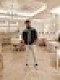Question: Encrypt "This is the final exam" with Playfair cipher using key "Guidance". Explain the steps involved.
0
mumbai university • 456 views
 modified 5 months ago  • written 5 months ago byneelchheda45 • 20
0
• The secret key in playfair cipher is made of 25 alphabet letters arranged in a 5 X 5 matrix in which I & J are considered the same when encrypting
• Different arrangements of the letters in the matrix can create many different secret keys
G U I/J D A
N C E B F
H K L M O
P Q R S T
V W X Y Z

The cipher uses three rules of encryption.

• If the two letters in a pair are located in the same row f the secret key , the corresponding encrypted character for each letter is the next letter to the right in the same row.
• If the two letters in a pair are located in the same column of the secret key, the corresponding encrypted characters for each letter is the letter beneath in the same column.
• If the two letters in a pair are hot located in the same column or row of the secret key, the corresponding encrypted characters for each letter is a letter that is in its own row but in the same columns as the other letter.

$P=P_{1} P_{2} P_{3} \qquad C=C_{1} C_{2} C_{3}$ $k=\left[\left(k_{1}, k_{2}\right)\left(k_{3}, k_{4}\right)\right]$

encryption : Ci = Ki Decryption : Pi = Ki

Thus

plaintext: " This is the final exam "

cipher : th is th ef in al ex am

Ciphertent by using all rules

'Th' = OP ..... by rule 3

'is' = DR ...... by rule 3

'ef = BN ..... by rule 2

'in' = FG ..... by rule 3

'al' = OI ..... by rule 3

'ex' = LI ...... by rule 2

'am' = DO ..... by rule 3

Thuswritten 5 months ago byneelchheda45 • 20